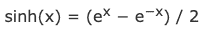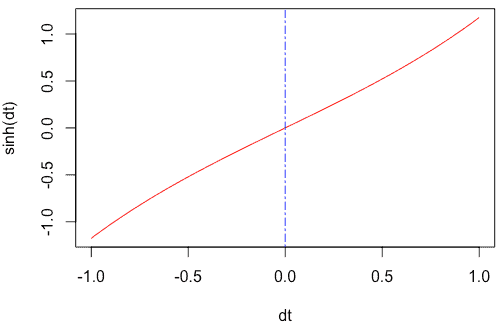# sinh in R: How to Use sinh() Function in R

The hyperbolic functions are analogs of the circular function or the trigonometric functions. The hyperbolic function occurs in the solutions of linear differential equations, calculation of distance and angles in the hyperbolic geometry, Laplace’s equations in the cartesian coordinates.

## Equation of sinh() function

The equation of the sinh() function is the following.The hyperbolic functions are defined within the algebraic expressions, including the exponential function (e^x) and its inverse exponential functions (e^-x), where e is the Euler’s constant.

## sinh in R

The sinh() is a built-in R function that takes a numerical value as an argument and returns the hyperbolic sine value of the numeric value. To calculate the hyperbolic sine value of the numeric value in R, use the sinh() function.

### Syntax

``sinh(x)``

### Parameters

x: It is a numeric value.

### Example

``````sinh(0)
sinh(1)``````

#### Output

`````` 0
 1.175201``````

## Calculating sinh() of complex number

Define a complex value and pass that value to the sinh() function.

``````d <- 5 + 1i
sinh(d)``````

#### Output

`` 40.09217+62.44552i``

## Plotting the sinh() function to a graph

We will use the seq() function to create a series of values and pass that to the plot() function, which will create a line chart using the sinh() function.

``````dt <- seq(-1, 1, by = 0.05)
plot(dt, sinh(dt), typ = "l", col = "red")
abline(v = 0, lty = 6, col = "blue")``````

#### Output## Applying sinh() function to a Vector

To create a Vector in R, use the c() function. Then pass that vector to the sinh() function.

``````rv <- c(-1, 0.5, 0, 0.5, 1)
sinh(rv)``````

#### Output

`` -1.1752012 0.5210953 0.0000000 0.5210953 1.1752012``

## Passing a pi to the sinh() function

The pi is an inbuilt constant in R programming, and its value is 3.141593.

Let’s find the pi constant’s sinh() value.

``sinh(pi)``

#### Output

`` 11.54874``

Let’s see another example of pi.

``sinh(pi / 4)``

#### Output

`` 0.868671``

That is it for the sinh() function in R programming.